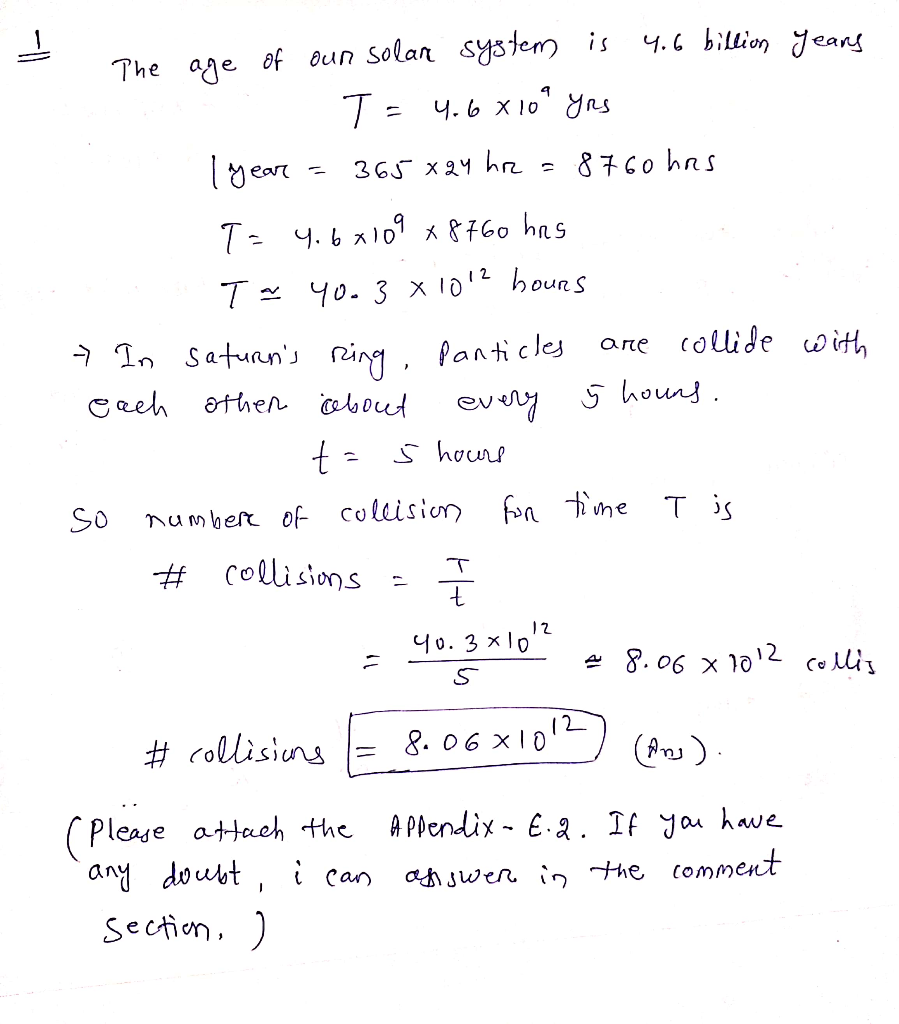# If a ring particle survived for the age of the solar system. how many collisions would it undergo?

1. Collisions in Saturn’s Rings : Each of the particles in the
densest part of Saturn’s rings collides with another particle about
every 5 hours. If a ring particle survived for the age of the solar
system, how many collisions would it undergo ? 2. Orbital Resonances. Using data in Appendix E.2, identify the
orbital resonance relationship between Saturn’s moon Titan and
Hyperion. [Recall that an orbital resonance means that if one
moon’s period times X (where X is an interger) equals the other
moon’s period times Y (where Y is an interger)—then the moons are
said to be in an X :Y resonance. For example, if the orbital period
of one moon were 1.5 times the orbital period of another moon, 1.5
is 3 divided by 2, and so the moons would be in a 3 to 2 (3 :2)
orbital resonance.] 3. We have a Jupiter: Suppose that our Solar System never had
the planet Jupiter. Describe at least three ways in which a
Jupiter-less solar system would be different from our current solar
system. In each case, CLEARLY EXPLAIN WHY 4. Where comets come from: Explain in your own words how and why
there are two different reservoirs of comets. Be sure to discuss
where the two groups of comets formed and what kinds of orbits they
travel on.

– The age of our solar system is 4.6 billion years T = 4.6 xlo” yrs 1 year = 365×24 hr = 8760 hrs T = 4.6×109 x 8760 hrs T = 40.3 81012 hours – In saturn’s ring, panticles are collide with each other about every 5 hours. t= 5 hours so number of collision for time T is # Collisions – I 40.3×10!? = 8.06 x 1012 collis # collisions E 8.06×1013) (Pony). (Please attach the Appendix – E.2. If you have any doubt i can assswer in the comment section )